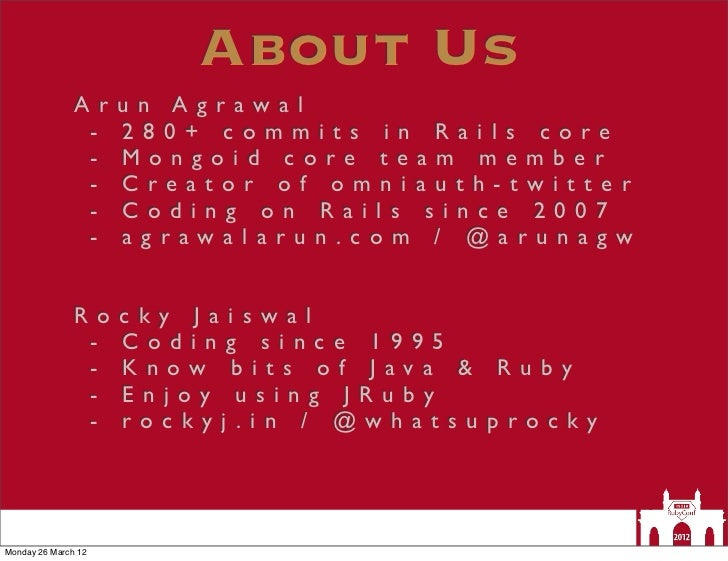Successfully reported this slideshow.Upcoming SlideShare
×

# About Us A r u When Ruby Meets Java - The Power of Torquebox

10,281 views

Published on

About Us A r u n A g r aw a l - 2 8 0 + c o m m i t s i n R a i l s c o re - M o n g o i d c o re t e a m m e m b e r - C re a t o r o f o m n i a u t h - t w i t t e r - C o d i n g o n R a i l s s i n c e 2 0 0 7 - a g r aw a l a r u n . c o m / @ a r u n a g w R o c k y J a i s w a l - C o d i n g s i n c e 1 9 9 5 - K n o w b i t s o f J av a & R u b y - E n j oy u s i n g J R u b y - ro c k y j . i n / @ w h a t s u p ro c k yMonday 26 March 12

Published in: Technology, Education
• Full Name
Comment goes here.

Are you sure you want to Yes No